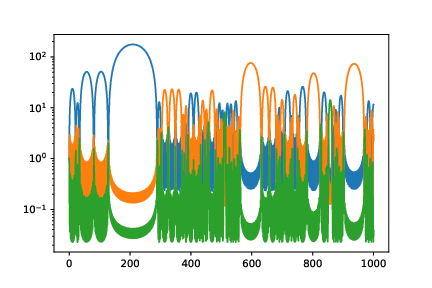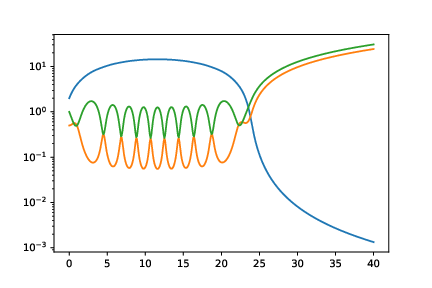# Higher dimensional affine fluids and geodesics of $$\mathsf{SL}(n)$$

2021 Conference on Geometric Analysis and Hyperbolic Equations — Guangxi University, Nanning, China — 2021.12.8

## Higher dimensional affine fluids and geodesics of $$\mathsf{SL}(n)$$

Willie Wai Yeung Wong
wongwwy@math.msu.edu
Michigan State University
2021 Conference on Geometric Analysis and Hyperbolic Equations

Slides available :   https://slides-n-notes.qnlw.info

## Credits page

• Joint work with:
• Audrey Rosevear, Amherst College
• Samuel Sottile, Michigan State University
• Support by NSF through the SURIEM (2020) summer REU site at MSU.
• Paper appears in La Matematica (2021)

## Outline

1. From Fluids to Geometry
2. Preliminary Analyses
3. Prior Results
4. Our results

## Euler-Arnol'd Equations

Fix a compact Riemannian manifold $(M,g)$. Denote by $\mathrm{Sdiff}(M)$ the set of all volume-preserving diffeomorphisms of $M$.

In Lagrangian coord., incompressible flow $\quad\cong\quad$ path $t\to \Phi_t$ in $\mathrm{Sdiff}(M)$.

Action (no potential energy, purely kinetic): $S[\Phi] = \int_0^T \int_M g|_{\Phi_t(y)}(\partial_t \Phi_t(y), \partial_t \Phi_t(y)) ~dy~dt.$ Inner integral defines a Riemannian metric on $\mathrm{Sdiff}(M)$.
Tangent space $\cong$ divergence-free vector fields; ELE are the Euler Equations

## Free Boundary

• Initial fluid domain $\Omega_0\subset \mathbb{R}^n$, smooth boundary; $\Omega_t$ later time slice
$\bar\Omega$ space-time domain
• Lagrangian / geodesic formulation (?)
• EoM should become incompressible Euler with boundary conditions:
$\begin{gathered} \partial_t u + u \cdot \nabla u = - \nabla p \newline \nabla\cdot u = 0 \newline p|_{\partial(\Omega_t)} = 0 \newline (1,u) \parallel \partial\bar{\Omega}\end{gathered}$

## Affinely constrained flows

• $\Omega_t = \Phi_t \Omega_0 = \gamma(t) + A(t) \cdot \Omega_0$
• $\gamma:\mathbb{R}\to \mathbb{R}^n$
• $A:\mathbb{R}\to \mathsf{SL}(n)$    (i.e. $$A\in \mathsf{GL}(n)$$ and $$\det(A) = 1$$)
• WLOG assume center of mass $\int_{\Omega_0} x ~dx = 0$
• Same action: reduce to $\begin{gathered} S = \int \left[ \mathrm{vol}(\Omega_0) |\dot{\gamma}(t)|^2 + \mathrm{tr}\left( \dot{A}(t) I_{\Omega_0} \dot{A}(t)^{\mathsf{T}} \right) \right]~dt \newline I_{\Omega_0} = \int_{\Omega_0} y y^T ~ \mathrm{d}y \end{gathered}$

## Geodesic motion on $\mathsf{SL}(n)$

$\begin{gathered} S = \int \left[ \mathrm{vol}(\Omega_0) |\dot{\gamma}(t)|^2 + \mathrm{tr}\left( \dot{A}(t) I_{\Omega_0} \dot{A}(t)^{\mathsf{T}} \right) \right]~dt \newline I_{\Omega_0} = \int_{\Omega_0} y y^T ~ \mathrm{d}y \end{gathered}$

• Product Riemannian metric on $\mathbb{R}^n\times \mathsf{SL}(n)$:
given $$v,w\in \mathsf{T}_p\mathbb{R}^n\cong \mathbb{R}^n$$ and $$\alpha,\beta \in \mathsf{T}_A\mathsf{SL}(n)\cong \mathbb{R}^{n^2}$$ $\langle (v,\alpha),(w,\beta)\rangle = v\cdot w + \mathrm{tr}(\alpha I_{\Omega_0} \beta^{\mathsf{T}}).$
• $$S$$ is the energy functional for paths on this Riemannian manifold.
• Center of mass motion splits from rotation/deformation.

## When is a constraint not a constraint?

• ELE of constrained motion generally has additional Lagrange multiplier term.
• Multiplier vanishes if unconstrained flow is tangent to constraint surface.

## Summary

• Treat $\mathsf{SL}(n)$ as submanifold of the Euclidean space $M(n)$ of $n\times n$ matrices with Hilbert-Schmidt metric $\langle \alpha,\beta\rangle = \mathrm{tr}(\alpha\beta^{\mathsf{T}})$.
• Geodesics are solutions to free boundary fluid flow, with $\Omega_0$ round ball.

## Discrete symmetry

• $$A \to A^{\mathsf{T}}$$ is isometry of $$M(n)$$, and fixes $$\mathsf{SL}(n)$$, so is isometry on $$\mathsf{SL}(n)$$.
• Notations
• $S(n)$: symmetric $n\times n$ matrices
• $\mathring{S}_+(n)$: positive definite elements of $\mathsf{SL}(n)\cap S(n)$
• Lemma. $S(n)\cap \mathsf{SL}(n)$ is totally geodesic in $$\mathsf{SL}(n)$$.
• Corollary. $\mathring{S}_+(n)$ is totally geodesic in $$\mathsf{SL}(n)$$.

## Continuous symmetries

• $$\mathsf{SO}(n)$$ acting on $$M(n)$$ by matrix multiplication is isometric & fixes $$\mathsf{SL}(n)$$

## Polar decomposition

Every $A\in \mathsf{SL}(n)$ has a unique factorization as $OP$ (or $PO$): $\begin{cases} O\in \mathsf{SO}(n) \newline P\in \mathring{S}_+(n)\end{cases}$

$\mathsf{SL}(n)$ foliated by cosets

So the geodesics are easy to describe, right?

Problem: Left actions generate right-invariant vector fields and vice versa

... so even with our conserved quantities, the motions on the two factors do not split.

## Some definitions

### Throat

Call $\mathsf{SO}(n)\subsetneq \mathsf{SL}(n)$ the "throat": it describes the points closest to the origin in $M(n)$.

### Second fundamental form

Sign convention: $\mathrm{II}(X,X) \gt 0$ if it curves away from the origin.

Second f.f. defined via normal pointing away from origin of $$M(n)$$.

## Geodesic equation

Let $X,Y\in \mathsf{T}_A \mathsf{SL}(n)$, then $\mathrm{II}(X,Y) = \frac{\mathrm{tr}(A^{-1}X A^{-1}Y)}{|A^{-1}|}$ and the geodesic equation reads $\ddot{A} = \frac{ \mathrm{tr}( A^{-1} \dot{A} A^{-1} \dot{A})}{\mathrm{tr}(A^{-1} A^{-T})} A^{-T}.$

## $n = 2$ is special: analysis

• Motion is completely integrable: $\dim(\mathsf{SL}(2)) = 3$ and conservation of
• Energy
• Angular momentum
• Vorticity
• Used in Roberts-Shkoller-Sideris (CMP 2020) where geodesics are fully classified.

## $n = 2$ geodesic classification

• The only bounded geodesic orbits the throat
• There exists semi-bounded geodesics converging to the throat
• All unbounded geodesics are "asymptotically linear" ($\mathrm{II}(\dot{\gamma},\dot{\gamma}) \to 0$)

## $n = 2$ is special: geometry

• $\mathsf{SL}(2)$ is a warped product $\mathring{S}_+(2) \times_f \mathbb{S}^1$
• Use the composite $A\mapsto OAO$ action
• double cover of $\mathsf{SL}(2)$ by $\mathring{S}_+(2) \times_f \mathsf{SO}(2)$.
• Use $\mathsf{SO}(2) = \mathsf{U}(1)$ and "take square roots"

## $n = 3$ prior results

Analyses of some special explicit solutions and their asymptotics

Sideris (ARMA 2017)

## $n$-independent prior results

• Virial Identity: let $A: t\to M(n)$ a $\mathsf{SL}(n)$-geodesic $\frac{d^2}{dt^2} |A|^2 = \frac{|\dot{A}|^2}{|A|} + \frac{n}{|A|^2} \mathrm{II}(\dot{A}, \dot{A}).$
• Proposition: if additionally $A$ is such that $\mathrm{II}(\dot{A}, \dot{A}) \gt 0$ for all $t \gt t_0$, then $A$ is unbounded and asymptotically linear.

Sideris (ARMA 2017)

## Significance of $\mathrm{II}(\dot{A}, \dot{A}) \gt 0$

• Rayleigh-Taylor Stability Condition: pressure decreases to zero as one approaches boundary
• Related to ill-posedness of full free boundary problem; Ebin (CPDE 1987)
• Contrapositive: any bounded geodesic corresponds to unphysical solution to free boundary fluid flow.

## Fluid Questions

• Ebin's example of illposedness is the $n = 2$ throat rotation.
• Unstable within class of affine flows
• What about higher dimensions? How much of the classification theorem persist?

## Higher dimensions are more curvy

• Theorem. $\mathsf{SL}(2)$ has bounded sectional curvature.

## Linear solutions are linearly stable

• Linear solutions: those whose images are lines in $M(n)$.
All in the form of $A(t) = A_0(\mathrm{Id} + t M)$ where $M$ nilpotent.
• Linear stability: Jacobi fields along such curves grow at most linearly.

## Bounded geodesics abound in $n \geq 4$

• Theorem. When $n = 2m \geq 4$, for every $\eta \geq |\mathrm{Id}|$, there exists a bounded geodesic $A(t)$ with $|A(t)| \equiv \eta$.Numerical simulation for $n = 6$, for generic data when axes are paired; plots are the three semi-major axial lengths.

## Refinement of Virial argument

$\frac{d^2}{dt^2} |A|^2 = \frac{|\dot{A}|^2}{|A|} + \frac{n}{|A|^2} \mathrm{II}(\dot{A}, \dot{A}).$

Lemma. When $n = 2$, we have $\frac{d^2}{dt^2} |A(t)|^2 \leq 0 \implies |A(t)| = |\mathrm{Id}|$.

Corollary. All geodesics except for the throat rotation are unbounded.

Emphatically false when $n \geq 3$.

## Virial revisited

Theorem. [sufficient conditions for unboundedness]

1. Solution is tangent to $\mathring{S}_+(n)$ (or another coset)
2. Solution has vanishing total angular momentum
3. Solution has vanishing total vorticity

## New formulation of equations

Given solution $A(t)$, define $\beta = A^{-1}A^{-\mathsf{T}}, \quad \omega = A^{\mathsf{T}} \dot{A} + \dot{A}^{\mathsf{T}} A, \quad \zeta = A^{\mathsf{T}} \dot{A} - \dot{A}^{\mathsf{T}} A$

• $\beta$: "square" of the "radial component" in polar decomposition
• $\omega$: "radial" velocity
• $\zeta$: "angular" velocity

Above is the vorticity version; angular momentum version moves the transpose to the other factor.

Cannot do both simultaneously.

## New formulation of equations

Solves problem with Killing vector field not pointing in the fibre direction.

\begin{align*} \frac{d}{dt} \beta & = -\beta \omega \beta \newline \frac{d}{dt}\omega & = \frac12(\omega + \zeta)^{\mathsf{T}}\beta(\omega + \zeta) + \underbrace{\frac{\mathrm{tr}\omega\beta\omega\beta}{2\mathrm{tr}\beta}}_{\geq 0} I + \underbrace{\frac{\mathrm{tr}\zeta\beta\zeta\beta}{2\mathrm{tr}\beta}}_{\leq 0} I \newline \frac{d}{dt}\zeta &= 0 \end{align*}

Compatibility condition: $\quad \mathrm{tr}\beta\omega = 0$.

Preserves "block diagonal structure"

## Block diagonal solutions

Assume $\beta, \omega,\zeta$ decompose into $2\times 2$ blocks (with one $1\times 1$ block when $n$ is odd) along the diagonal.

### A boundedness criterion

Theorem. Let $n$ be even. If the initial data of $\beta,\omega$ are built from $2\times 2$ blocks that are pure-trace, and if $\zeta$ has no vanishing bocks, then the solution is bounded.

When $n = 2$, hypothesis only possible with rotation at throat.

## "Swirling and shear flows"

Theorem. If the initial data has the form $\beta_0 = \begin{pmatrix} b_1 \mathrm{Id}_{2m} \newline & b_2 \mathrm{Id}_{n - 2m}\end{pmatrix}, \quad \omega_0 = \begin{pmatrix} w_1 \mathrm{Id}_{2m} \newline & w_2 \mathrm{Id}_{n-2m} \end{pmatrix}$ and writing $\varepsilon$ for the antisymmetric $2\times 2$ matrix $\zeta_0 = \begin{pmatrix} z (\underbrace{\varepsilon \oplus \cdots \oplus \varepsilon}_{m \text{ copies}}) \newline & 0 \end{pmatrix};$ then the corresponding solution is unbounded, and asymptotically linear.

Sideris (ARMA 2017) described $n = 3$ and $m = 1$ with explicit integration

## Further generalization?Numerical simulation of a generalized swirling and shear flow with three sets of axes, instead of two.

## Application: Asymptotically linear solutions can be unstable

• Let $A(t)$ be a swirling and shear flow: we can show that $\mathrm{II}(\dot{A}, \dot{A})$ is eventually positive.
• So $A(t)$ is "asymptotically linear"

## Implication

• When $n = 2$, unbounded geodesics are stable (in a sense), with perturbations still generating physical solutions (satisfying Rayleigh-Taylor).
• When $n = 2m \geq 4$, there exists unbounded physical solutions that appear as limits of bounded non-physical solutions.

## Further questions

• Conjecture. When $n$ is odd, $\mathsf{SL}(n)$ has no bounded geodesics.
• Conjecture. The only solutions with $|A(t)|$ constant are those we found.
• Question. Do there exist unbounded geodesics which are not asymptotically linear when $n \gt 2$?